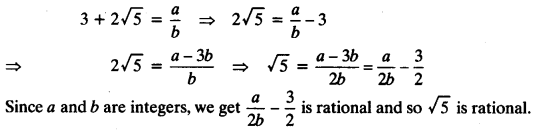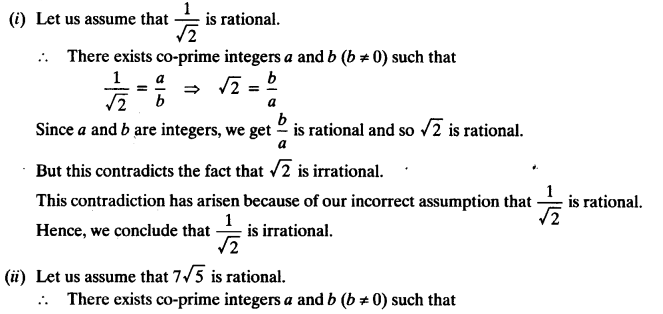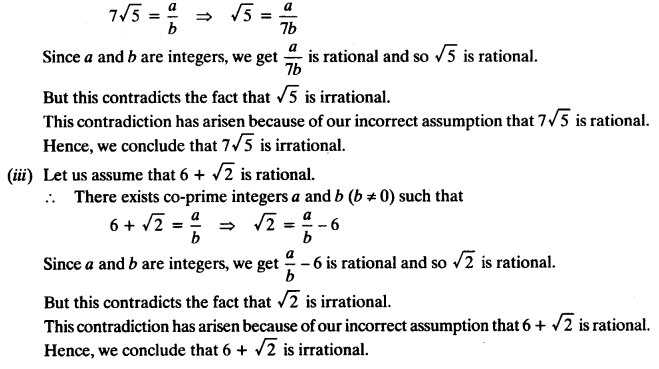NCERT Solutions for Class 10 Mathematics Chapter 1 Real Numbers Ex 1.3 are part of NCERT Solutions for Class 10 Maths. Here we have given NCERT Solutions for Class 10 Mathematics Chapter 1 Real Numbers Ex 1.3.

 Board CBSE Textbook NCERT Class Class 10 Subject Maths Chapter Chapter 1 Chapter Name Real Numbers Exercise Ex 1.3 Number of Questions Solved 3 Category NCERT Solutions

### NCERT Solutions for Class 10 Maths Chapter 1 Real Numbers Ex 1.3

Question 1.
Prove that √5 is irrational.
Solutions:
Let us assume that is rational.
∴ There exists co-prime integers a and b (b ≠ 0) such that
√5 = $$\frac { a }{ b }$$ ⇒ √5b= 0
Squaring on both sides, we get
5b2= a2…… (i)
⇒ 5 divides a2 ⇒ 5 divides a
So, we can write a = 5c for some integer c.
From (i) and (ii)
5b2 = 25c2
⇒ b2 = 5c2
⇒ 5 divides b2
⇒ 5 divides b
∴ 5 is a common factor of a and b.
But this contradicts the fact that a and b are co-primes.
This contradiction has arisen because of our incorrect assumption that √5 is rational.
Hence, √5 is irrational.

Question 2.
Prove that 3 + 2√5 is irrational.
Solutions:
Let us assume that 3 + 2√5 is rational.
∴ There exists co-prime integers a and b(b ≠ 0) such thatBut this contradicts the fact that √5 is irrational.
This contradiction has arisen because of our incorrect assumption that 3 + 2√5 is rational. Hence, we conclude that 3 + 2√5 is irrational.

Question 3.
Prove that the following are irrationals.Solutions:We hope the NCERT Solutions for Class 10 Mathematics Chapter 1 Real Numbers Ex 1.3 help you. If you have any query regarding NCERT Solutions for Class 10 Mathematics Chapter 1 Real Numbers Ex 1.3, drop a comment below and we will get back to you at the earliest.